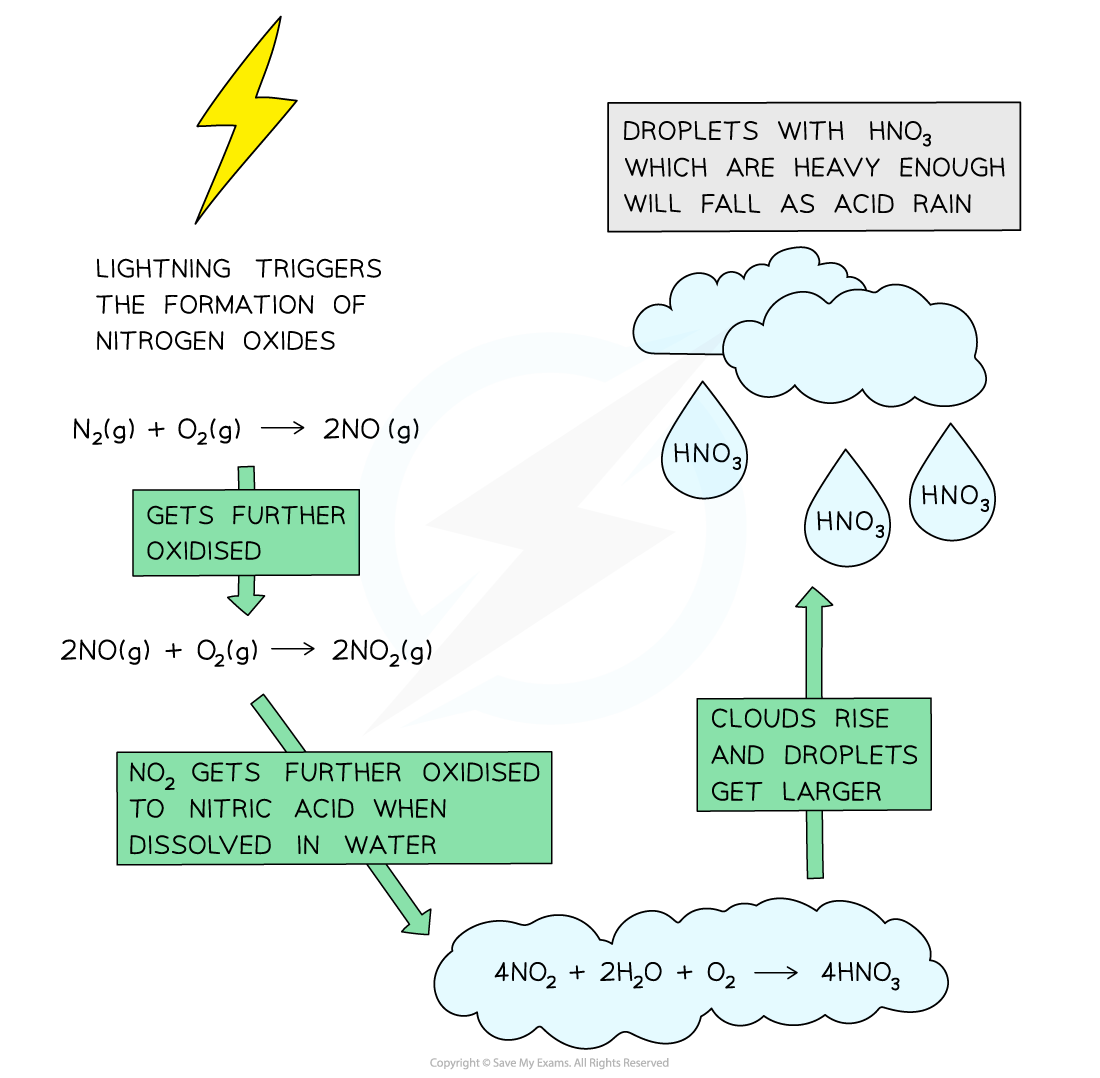# IB DP Chemistry: SL复习笔记8.3.1 Acid Deposition

### Acid Deposition

#### What is acid deposition?

• Rain is naturally acidic because of dissolved CO2 which forms carbonic acid

H2O (l) + CO(g)  ⇌  H2CO(aq)

• Carbonic acid is a weak acid and dissociates in the following equilibrium reaction giving a pH of 5.6

H2CO(aq)  ⇌  H+ (aq) +  HCO3(aq)

• For that reason acid rain is defined as rain with a pH of below 5.6
• Acid deposition includes all processes by which acidic components leave the atmosphere
• This could be gases or precipitates
• There are two types of deposition: wet acid deposition and dry acid deposition
• Wet acid deposition refers to rain, snow, sleet, hail, fog, mist and dew
• Dry acid deposition refers to acidic particles and gases that fall to the ground as dust and smoke
• Acid deposition is formed when nitrogen or sulfur oxides dissolve in water to form HNO3, HNO2, H2SO4 and H2SO3

### Acid Deposition Equations

#### Formation of sulfur based acids

• Fossil fuels are often contaminated with small amounts of sulfur impurities
• When these contaminated fossil fuels are combusted, the sulfur in the fuels get oxidised to sulfur dioxide

S (s) + O(g) → SO(g)

• Sulfur dioxide may be further oxidised to sulfur trioxide

2SO(g) + O(g) ⇌ 2SO(g)

• The sulfur dioxide and sulfur trioxide then dissolve in rainwater droplets to form sulfurous acid and sulfuric acid

SO2(g) + H2O  (l) → H2SO(aq)

SO(g) + H2O (l) → H2SO(aq)

• These acids are components of acid rain which has several damaging impacts on the environment

#### Formation of acid rain by nitrogen oxides

• The temperature in an internal combustion engine can reach over 2000 °C
• Here, nitrogen and oxygen, which at normal temperatures don’t react, combine to form nitrogen monoxide:

N(g)+ O(g) ⇌ 2NO (g)

• Nitrogen monoxide reacts further forming nitrogen dioxide:

2NO (g) + O(g) ⇌ 2NO(g)

• Nitrogen dioxide gas reacts with rain water to form a mixture of nitrous and nitric acids, which contribute to acid rain:

2NO(g) + H2O (l)  → HNO(aq) + HNO(aq)

• Lightning strikes can also trigger the formation of nitrogen monoxide and nitrogen dioxides in air
• Nitrogen dioxide gas reacts with rain water and more oxygen to form nitric acid

4NO(g) + 2H2O (l) + O(g)→ 4HNO(aq)

• When the clouds rise, the temperature decreases, and the droplets get larger
• When the droplet containing these acids are heavy enough, they will fall down as acid rainThe diagram shows the formation of acid rain by the oxidation of nitrogen dioxide